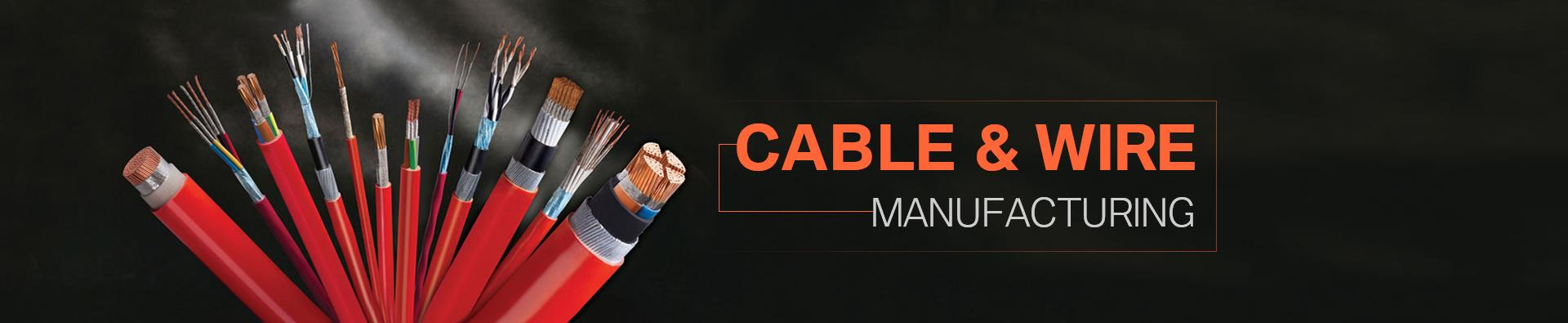Categories

Office Mob: +8615524105871

Office Tel: +86-024-31931990

Office Fax: +86-024-22845391

E-mail: info@eoexport.com

Knowledge
Home > Knowledge > Content
Output calculation and length conversion of copper wire drawing and stranded wire, calculation formula of output of annealing machine
Apr 19, 2018

Copper wire Drawing output Calculation formula:

Line diameter (mm) x-ray (mm) x0.7854x copper density X-ray velocity (m/min) x-minute ÷1000=kg (theoretical output)

Calculation formula of copper annealing yield:

Line diameter (mm) x-ray (mm) x0.7854x copper density X-ray velocity (m/min) x-minute X-root ÷1000=kg (theoretical output)

Calculation formula of copper stranded wire output:

Line diameter (mm) x-ray (mm) x0.7854x copper density X-stranded x2x strip X-ray Velocity (m/min) x-min ÷1000=kg The total diameter of multiple copper strands after the calculation formula:

Total diameter of x1.155x line diameter +0.1=

Copper wire Drawing-calculation of the length and weight of the relationship:

Weight = length x copper density x0.7854x line diameter X-ray ÷1000. According to this formula, the actual length of a coil can be deduced. This has practical value in production. For example, a small pull production of 0.12MM diameter, 300 sets, to set how much length is suitable?

Using this formula can be calculated.

Calculation of length and weight of copper stranded wire: Weight = length x7.19x line diameter X-ray X-root number ÷1000.

• Categories